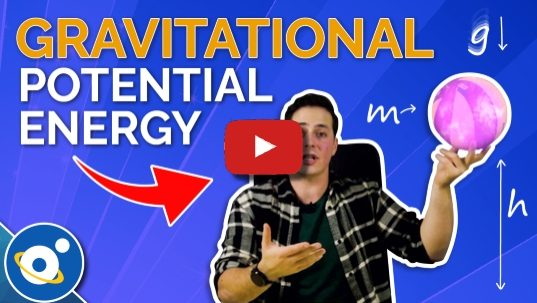# Potential Energy Calculator

Created by Mateusz Mucha
Reviewed by Jack Bowater
Last updated: Apr 13, 2022

This potential energy calculator enables you to calculate the stored energy of an elevated object. The full name of this effect is gravitational potential energy because it relates to the energy which is stored by an object as a result of its vertical position or height.

Prefer watching rather than reading? Check out our video lesson on gravitational potential energy here:• How to calculate potential energy
• What the gravitational potential energy formula is

## How to calculate potential energy

The easiest way to calculate gravitational potential energy is to use our potential energy calculator. This tool estimates the potential energy on the basis of three values. These are:

1. The mass of the object;
2. Gravitational acceleration, which on Earth amounts to 9,81 m/s² or 1 g; and
3. The height of the object.

Then the calculator will give you the result in joules. As with all of our calculators, this potential energy calculator does not have to be exclusively used to calculate potential energy. Just input any three of the four variables to find the fourth with ease.

If you want to calculate the energy of an object which is in motion, our kinetic energy calculator is highly recommended.

## The potential energy formula

Let's look under the hood of the potential energy calculator. To help you picture it, our example will be the massive wrecking ball on a crane. The gravitational potential energy of this ball depends on two factors - the mass of the ball and the height it's raised to. The relationship between gravitational potential energy and the mass and height of an object is described by the following equation:

PE grav. = m × h × g

Where:

• PE grav. - Gravitational potential energy of an object;
• m - Mass of the object in question;
• h - Height of the object; and
• g - Gravitational field strength acting upon the object (1 g or 9.81 m/s2 on Earth).

The formula is relatively simple. An object which is not raised above the ground will have a height of zero and therefore zero potential energy. When you double the mass or the height of an object, its potential energy will also double.

Mateusz Mucha
Mass
kg
Grav. acceleration
g
Height
m
Potential energy
J
People also viewed…

### Helium balloons

Wondering how many helium balloons it would take to lift you up in the air? Try this helium balloons calculator! 🎈

### Number density

Use this number density calculator to compute the charge carrier number density of specific conductors.

### Pump horsepower

Determine the hydraulic and shaft power of pumps using the pump horsepower calculator.

### Significant figures

The significant figures calculator performs operations on sig figs and shows you a step-by-step solution!